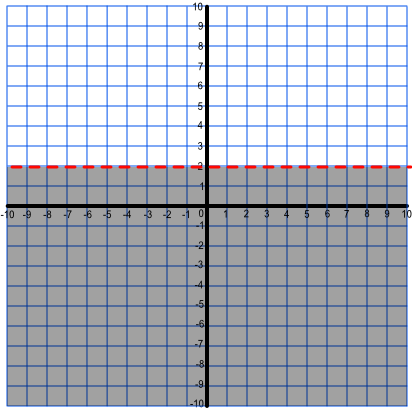Inequalities on a Graph

# Inequalities on a Graph

GCSE(H),

Inequalities can be represented on a graph. The inequality is shown by the line of the inequality, and a shaded area. The line of the inequality is shown in one of two ways:

• a solid line indicates that the line is included in the inequality: it is either ≤ or ≥;

• a dotted line indicates that the line is not included: it is either > or < .

## Examples

1. Show, on a graph, the inequality described by -3 < y ≤ 2.

Answer:Note that the line y = 2 is a solid line (≤), and that y = -3 is a dotted line (<)

2. What inequality does this graph describe? Write the inequality using set notation.Answer: {y: < 2}

The shaded area is less than 2: because the line is dotted, the value of y = 2 is not included.